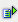The function trunc (num) returns the next smaller whole-numbered value of the value (num). Negative values are rounded in direction to 0.

Syntax: =trunc(num)Example =trunc(2.2) is 2 =trunc(-2,2) is -2